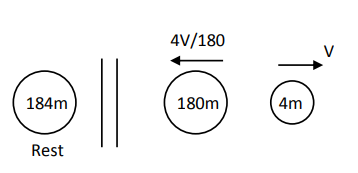# A nucleus with mass number 184 initially at rest emits an

Question:

A nucleus with mass number 184 initially at rest emits an $\alpha$-particle. If the $Q$ value of the reaction is $5.5 \mathrm{MeV}$, calculate the kinetic energy of the $\alpha-$ particle.

1. $5.0 \mathrm{MeV}$

2. $5.5 \mathrm{MeV}$

3. $0.12 \mathrm{MeV}$

4. $5.38 \mathrm{MeV}$

Correct Option: , 4

Solution:$\frac{1}{2}(4 \mathrm{~m}) \mathrm{v}^{2}+\frac{1}{2}(180 \mathrm{~m})\left(\frac{4 \mathrm{v}}{180}\right)^{2}=5.5 \mathrm{MeV}$

$\Rightarrow \quad \frac{1}{2} 4 \mathrm{mv}^{2}\left[1+45\left(\frac{4}{180}\right)^{2}\right]=5.5 \mathrm{MeV}$

$\Rightarrow \quad K \cdot E_{\alpha}=\frac{5.5}{1+45 \cdot\left(\frac{4}{180}\right)^{2}} \mathrm{MeV}$

$\mathrm{KEE}_{\alpha}=5.38 \mathrm{MeV}$